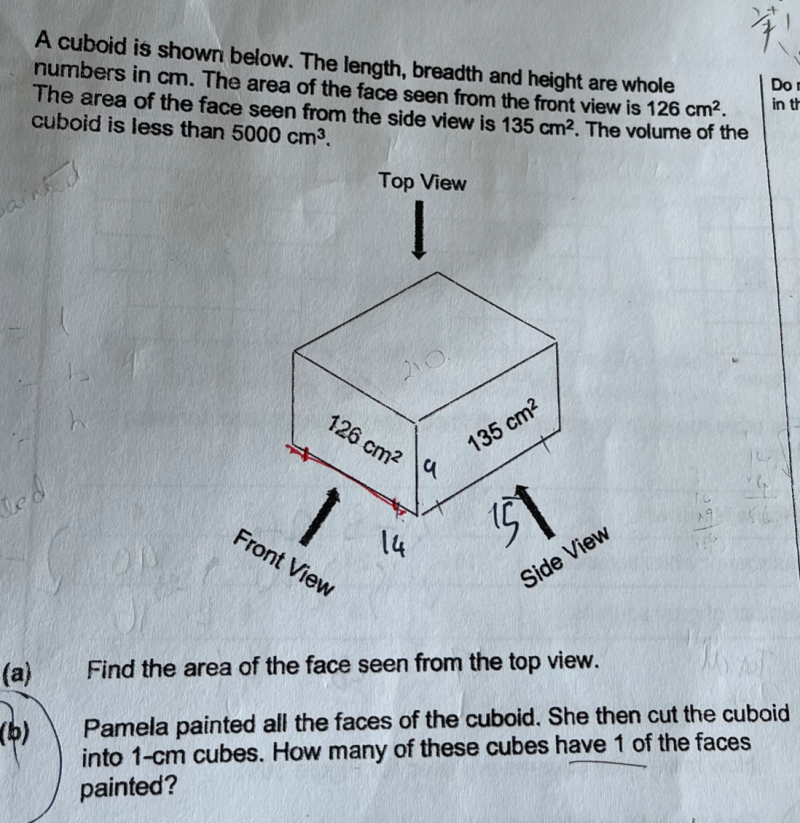# Question126                                                       135

/               \                                          /                 \

2                   63                                     5                  27

/          \                                                 /             \

3              21                                           3                   9

/        \                                                        /         \

3           7                                                               3

/      \                                                        /       \

7             1                                                3             1

(a) We can see that 3×3 = 9 is common to both factorisation which is the common height

and 3 albeit common is not chosen as the height because the volume will exceed 5000.  (42 x 45 x 3 = 5670)

So from 126  = 9 x 14        and          135 = 9 x 15

Alternatively, list the factors of both 126 and 135 as follows:

Factors of 126

1 x 126,

2 x 63,

3 x 42,

6 x 21,

7 x 18,

9 x 14

Factors of 135

1 x 135

3 x 45

5 x  27

9 x 15

There are 3 sets of factors with one common factor, namely, 1, 3 and 9 but 1 and 3 will have volume exceeding 5000 so 9 is chosen.

we get the length and breadth of the top view, hence area of top view = 14 x 15 = 210 cm2

(b)  Front view with cubes one-side painted = (7 x 12) x 2 = 168

Side view with cubes one-side painted  = ( 7 x 13) x 2  = 182

Top + bottom  view with cubes one side painted = (12 x 13) x 2 = 312

Hence total cubes with one-side painted = 168 + 182 + 312 = 662

1 Reply 1 Like